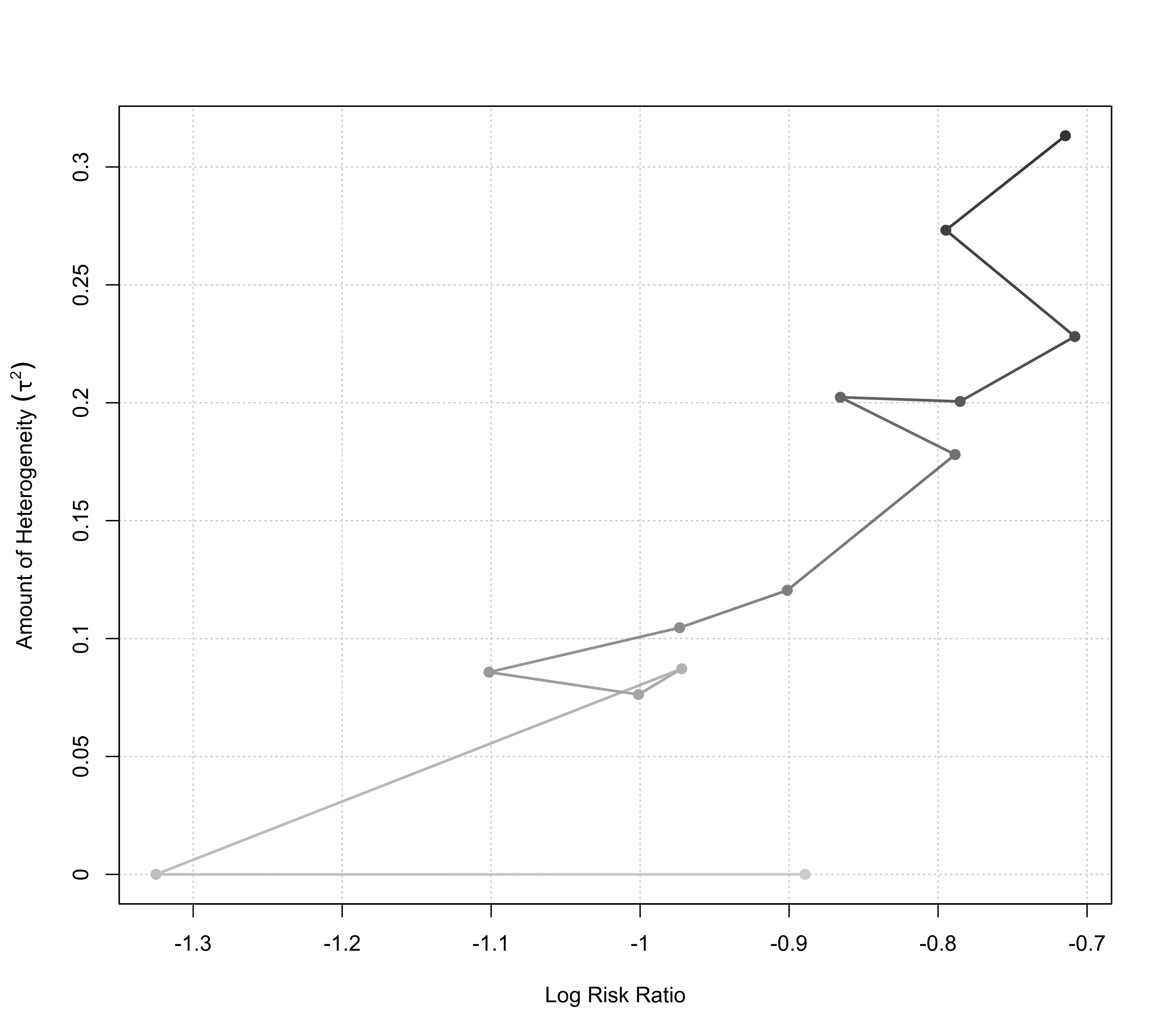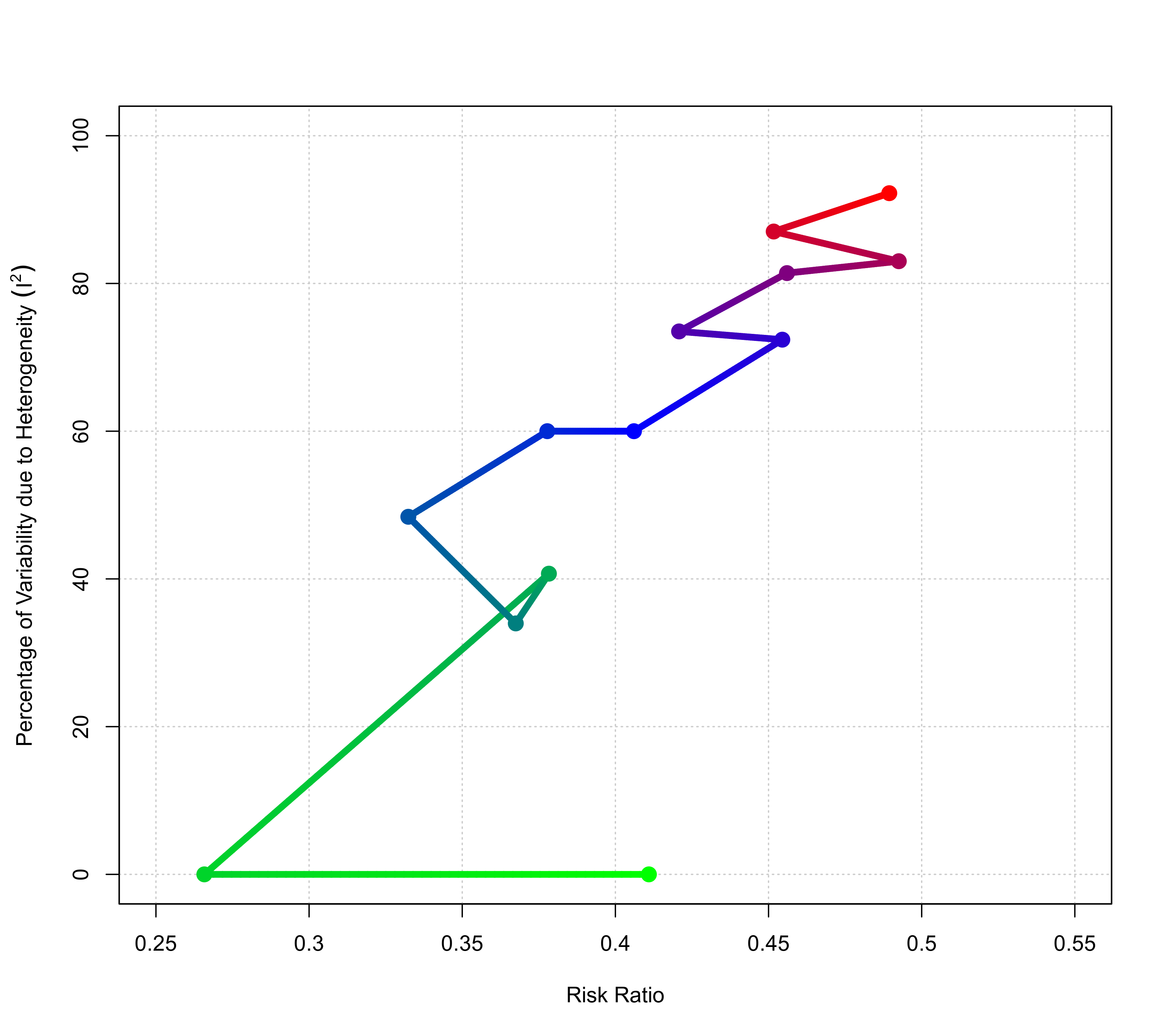Function to plot objects of class "cumul.rma".

# S3 method for cumul.rma
plot(x, yaxis, xlim, ylim, xlab, ylab,
at, transf, atransf, targs, digits, cols,
grid=TRUE, pch=19, cex=1, lwd=2, ...)

## Arguments

x

an object of class "cumul.rma" obtained with cumul.

yaxis

either "tau2", "I2", or "H2" to indicate what values should be placed on the y-axis. See ‘Details’.

xlim

x-axis limits. If unspecified, the function sets the x-axis limits to some sensible values.

ylim

y-axis limits. If unspecified, the function sets the y-axis limits to some sensible values.

xlab

title for the x-axis. If unspecified, the function sets an appropriate axis title.

ylab

title for the y-axis. If unspecified, the function sets an appropriate axis title.

at

position of the x-axis tick marks and corresponding labels. If unspecified, the function sets the tick mark positions/labels to some sensible values.

transf

optional argument to specify a function to transform the summary estimates (e.g., transf=exp; see also transf). If unspecified, no transformation is used.

atransf

optional argument to specify a function to transform the x-axis labels (e.g., atransf=exp; see also transf). If unspecified, no transformation is used.

targs

optional arguments needed by the function specified via transf or atransf.

digits

optional integer to specify the number of decimal places to which the tick mark labels of the x- and y-axis should be rounded. Can also be a vector of two integers, the first to specify the number of decimal places for the x-axis, the second for the y-axis labels (e.g., digits=c(2,3)). If unspecified, the function tries to set the argument to some sensible values.

cols

vector with two or more colors for visualizing the order of the cumulative results.

grid

logical to specify whether a grid should be added to the plot. Can also be a color name.

pch

plotting symbol to use. By default, a filled circle is used. See points for other options.

cex

symbol expansion factor.

lwd

line width.

...

other arguments.

## Details

The function can be used to visualize the results from a cumulative meta-analysis as obtained with the cumul function.

The plot shows the model estimate (i.e., the estimated overall/average outcome) on the x-axis and some measure of heterogeneity on the y-axis in the cumulative order of the results in the "cumul.rma" object. By default, $$\tau^2$$ is shown on the y-axis for a random-effects model and $$I^2$$ otherwise, but one can also use argument yaxis to specify the measure of heterogeneity to place on the y-axis.

The color gradient of the points/lines indicates the order of the cumulative results (by default, light gray at the beginning, dark gray at the end). A different set of colors can be chosen via the cols argument. See ‘Examples’.

## Author

Wolfgang Viechtbauer wvb@metafor-project.org https://www.metafor-project.org

cumul for the function to conduct a cumulative meta-analysis.

## Examples

### calculate log risk ratios and corresponding sampling variances
dat <- escalc(measure="RR", ai=tpos, bi=tneg, ci=cpos, di=cneg, data=dat.bcg)

### random-effects model
res <- rma(yi, vi, data=dat)

### cumulative meta-analysis (in the order of publication year)
sav <- cumul(res, order=year)

### plot of model estimate and tau^2 over time
plot(sav)### illustrate some other plot options
plot(sav, yaxis="I2", ylim=c(0,100), transf=exp, xlim=c(0.25,0.55),
lwd=5, cex=1.5, cols=c("green","blue","red"))